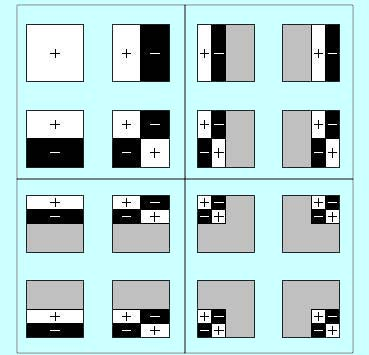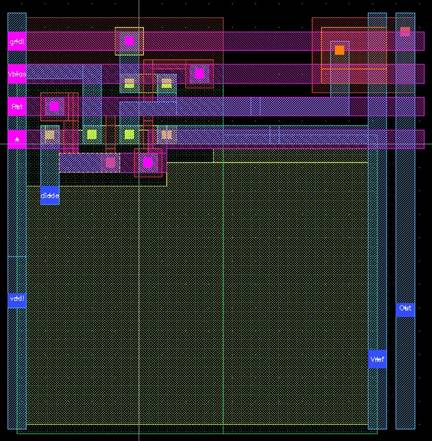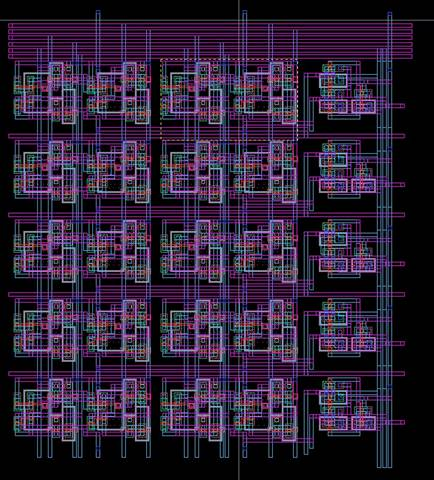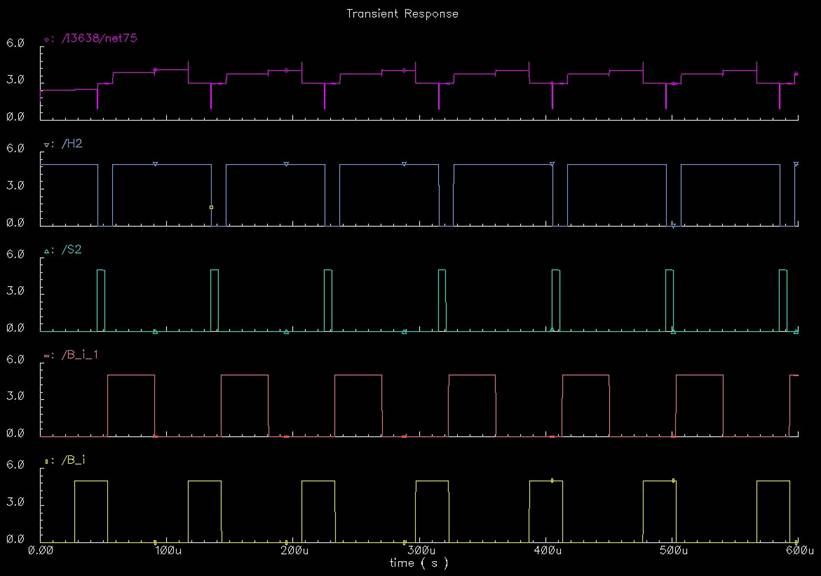# An Analog Wavelet Transform CMOS Imager Chip

spanda@bme.jhu.edu
vls@jhu.eduLayout of the entire chip with padframe included.

## Abstract

We present the design of a charge-mode CMOS Imager Chip which computes Discrete Wavelet Transform through focal plane image processing. The architecture uses Haar pyramid decomposition , which is the simplest tool for multi-resolution image analysis The  Haar computation can be realized as a 2-D Matrix Vector Multiplication problem , with multiplication achieved by presenting bit-serial inputs to a two-stage correlated double sampling (CDS) circuit. With the proposed architecture, it is also possible to compute other unitary image transforms like for example Walsh Transform and Hadamard Transform. In addition it can be used as a random access imager , where each pixel can be addressed independently. The entire chip is implemented on a 0.35um CMOS process with 16x24 active pixel sensors (APS) array. The initial prototype is laid out on a 1.5mmx1.5mm padframe.

Keywords: Focal plane image processing, Wavelet transform, Multi-resolution image analysis

## Theory

DWT extracts information from a signal at different scales. The first level of decomposition captures the highest frequency components of a signal, while the second and later decompositions extract progressively coarser level information (lower frequency components). In this way, it can be regarded as a filter bank with varying levels of resolution. Two filters that are needed here, one high-pass and one low-pass. A computationally efficient way to implement such a filter bank is to regard sums and differences as low-pass and high-pass filters. In this way, the output of a high-pass filter is the DWT coefficient while the output of the low-pass filter is fed to the next stage for higher-order coefficients, as shown in Figure 1.Figure 1: Basic pyramid decomposition Figure 2: Haar basis functions

Haar is the simplest transform for computing DWT. The haar algorithm takes sums and differences of pixels in a given image at different resolutions. As shown in the Figure 2, a family of Haar basis functions can be obtained by simply translating and dilating the mother wavelet.

 For a 2-D image, the Haar wavelet decomposition can be defined as a product of inner and outer coefficients i.e.: W (i,j) = (ΣA(i)×P(i,j))×B(j)   Where A(i)’s are inner product multiplicands, B(i)’s are outer product multiplicands and P(i,j) is the value at each pixel. In Haar, these multiplicands can assume three values: +1, 0 or -1. Therefore we are either adding all the pixel values, or taking their differences or not getting any contribution from them at all. For a 4×4 order image, the complete wavelet transform coefficients are shown in Figure 3.   The four pixels on the bottom left contain vertical edge information. Four pixels on the top right contain horizontal edge information and pixels at bottom right contain diagonal edge information. It also shows that the pixel that represents zero-frequency component (DC value) of the image is computed by simply adding all the pixels together. The next successive coefficients will involve summing and taking differences with finer resolution.Figure 3: Haar decomposition for a 4 x 4 image

## System Architecture

 The chip consists of three main components: a 16×24 pixel array, two correlated double sampling (CDS) stages and inner/outer product shift registers array at the periphery. Inner product coefficients are provided through rows and outer product coefficients are provided in columns. The first CDS bank is used to sum A(i)×P(i,j) across every columns. Each column has its own CDS and there are a total of 24 CDS circuits in the first stage. In the second CDS stage, charges across all columns are multiplied with Bi’s and their result added together. So only one CDS is needed in the second stage. Finally the output of this CDS is sent off-chip, as analog output. In this way, for one frame, the entire wavelet spectrum can be obtained in 16×24 clock cycles. Block diagram of the entire chip is shown Figure 4. A screenshot of the complete schematic is shown here.Figure 4: Top-level block diagram of the entire chip

The pixel circuit diagram and layout are shown in Figure 5. The capacitor used is of 20f F. It operates as a chare-mode device and therefore the voltage discharge from the APS is directly proportional to the light intensity. The capacitor in each cell is needed to sum all the charges across a column along a single wire. Inner product multiplicand, A is provided at the gate of M5. If A is kept low during pre-charge phase of CDS and high during evaluation phase, the APS dumps a charge -1)×P(i,j) on the common line. Likewise it dumps a charge P(i,j) if A is high in pre-charge and low during evaluation. No charge is dumped if A is held constant. Since, multiple APS cells are active at a time along a column, so a biasing transistor M2 has to be placed inside each cell. Each cell has a fill factor of approximately 60%, with dimensions of 89λ×89λ.Figure 5: Pixel circuit diagram and layout

The output of all the pixels along a column is sent to a correlated double sampling circuit. The CDS circuit is a basic sample and hold cell, and its layout is shown in Figure 6. CDS circuits are generally used in imagers to reduce the effect of fixed pattern noise. In this design, we are using CDS to compute difference of charge dumped across common line for two different phases of A. The capacitors used here are 320f F, because they need to store charge dumped by 16 cells. In order to get a high gain, we are using a cascode inverter instead of ordinary inverter. For the output, depending upon which phase B(i)’s are in, the output capacitor is either connected to CDS output or Vref. This is done during pre-charge/evaluation phase of the second CDS stage. Also worth noting is that width of CDS circuit is also 89λ, so it abuts exactly with the flip-flops.Figure 6: CDS circuit diagram and layout

There is some digital circuitry at the periphery of the chip which is mainly composed of an array of shift registers, for feeding different phases of A(i)’s and B(i)’s in a bit-serial fashion. The layout of the shift register had to be made very compact, since that was the main bottleneck in having high pixel density. Currently each flip flop fits inside 89λ×154λ. The dimension that is of more importance is 89λ, because it affects width and height of pixels and essentially the resolution of the imager. The clocks for these are generated through a simple circuit that generates two non-overlapping clocks, given one clock input. The schematic of each flip-flop is available here, and its layout is available here. The advantage of using row and column shift registers is that they take up far less area than decoder. In addition, the design is more scalable because in case of decoders the circuit complexity would increase exponentially. Schematic and layout of row and column shift registers is shown in Figure 7. Different phases of product coefficients are selected through multiplexors.

We need to store two phases of inner product coefficients and four phases of outer product coefficients. So there are two shift register arrays for rows and four shift register arrays for columns.Figure 7: Row and column shift registers

## Simulation Results

The simulation results obtained by simulating one pixel of the chip are shown in Figure 1. Here is the description of signals:

 net34 Output of one pixel. Voltage which represents the charge dumped by a cell (Figure 5) net74 Voltage across capacitor of CDS stage 1 (Figure 6) S1 Sample of stage 1 (Figure 6) H1 Hold of stage 1 (Figure 6) Rst Reset signal for the APS. Notice that in order to ensure that we experience same discharge across photodiode, we have to present two reset signals for both the phases of A (Figure 5) A Inner product coefficient (It goes from 0 to 1 so we are multiplying by -1) (Figure 5)

Here we are approximating a photocurrent of 60pA. All the clocks are operating at a frequency of 11 kHz. Sequencing of all the clocks is very important, so we should compute outer products only when we are in hold phase of inner product CDS circuit.Figure 8: Simulation results for a single pixel

The simulation trace obtained after simulating 4×4 pixel array is shown in Figure 9. Here is the description of signals:

 net75 Wavelet coefficient, voltage across capacitor of the second CDS circuit. It is valid only during the time when hold of the second stage is high H2 Hold of stage 2 S2 Sample of stage 2 B_i The component of outer product coefficient which controls CDS1 output connection to its output capacitor (Figure 6) B_i_1 Second component of B_i_1 which controls Vref connection to output connection

The outer product coefficients, Bi’s are presented as two components: one controls transmission gate which connects CDS stage 1 buffer to output capacitor. The second component controls transmission gate which connects Vref to output capacitor. The configuration shown in the diagram multiplies inner product with +1. If it was required to multiply with -1, we would have asserted both the components in opposite phases.Figure 9: Simulation results for a 4×4 array.

From simulation results, the range of operation of the output voltage was between approximately between 1.76 to 4.23 volts, with reference at 2.96 volts. The coefficients below reference indicate negative values. For further confirming that the chip is operating well, we covered some cases of adding and subtracting pixel values. The results were satisfactory, although small amount of error was observed. Our understanding is that it was due to charge injection in the APS cell.

## Pinout Assignments with Description

 `1.` `Vref_SH1` `CDS Stage1 reference voltage` `2.` `Vref_aps` `Reference voltage of pixel cell ` `3.` ` ` ` ` `4.` ` ` ` ` `5.` `gnd` `Ground` `6.` ` ` ` ` `7.` ` ` ` ` `8.` ` ` ` ` `9.` ` ` ` ` `10.` `A_phi1` `2nd phase value of inner product coefficient` `11.` `Rst` `Reset signal of APS cell` `12.` `Vbias` `Biasing voltage of APS cell` `13.` `A_sel` `Input to the multiplexor which selects current phase of inner product coefficient` `14.` `A_phi0` `1st phase value of inner product coefficient` `15.` `vdd` `Vdd` `16.` `VBP1_SH2` `Biasing voltage of cascade inverter, CDS 2nd stage` `17.` `VBP2_SH2` `Biasing voltage of cascade inverter, CDS 2nd stage` `18.` `VBN1_SH2` `Biasing voltage of cascade inverter, CDS 2nd stage` 19. S2 Sample of CDS 2nd stage 20. H2 Hold of CDS 2nd stage `21.` `Vref_SH2` `Reference voltage, CDS 2nd stage` `22.` `Wout` `Output (Wavelet coefficient)` `23.` `Prg_enb` `Controls shifts registers latching` `24.` Prg_clk Clock for programming shift registers 25. B_sel Selects phase of the outer product coefficients 26. B_zero Used to isolate CDS stage 1 from CDS stage 2 during precharge and evaluate of CDS stage 1 `27.` `Bi_Phi0` `1st phase of Bi` `28.` `Bi_phi1` `2nd phase of Bi` `29.` `Bi_1_phi0` `1st phase of Bi_1` `30.` `Bi_1_phi1` `2nd phase of Bi_1` `31.` `Phi_1_inv` `Output clock (for testing)` 32. Phi_1 Output clock (for testing) 33. Phi_2_inv Output clock (for testing) 34. 35. `36.` `H1` `Hold of CDS stage 1` `37.` `S1` `Sample of CDS stage 1` 38. VBN1_SH1 `Biasing voltage of cascade inverter, CDS 1st stage` 39. VBP2_SH1 `Biasing voltage of cascade inverter, CDS 1st stage` 40. VBP1_SH1 `Biasing voltage of cascade inverter, CDS 1st stage`

## References

   R. Gonzalez and R. Woods, “Digital Image Processing,” Addison-Wesley, 1992.

   R. Genov and G. cawenberghs, “Charge-mode parallel architecture for vector-matrix multiplication,” IEEE Transactions on Circuits and Systems-II, Analog and Digital Signal Processing, Vol. 48 pp 930-936, Oct. 2001.

   S. Decker, R. Mcgrath, K. Brehmer and C. Sodini, “A 256x256 CMOS imaging array with wide dynamic range pixels and column-parallel digital output,” IEEE Journal of Solid-State Circuits, vol. 33, pp 2081-2090, Dec 1998.

   O. Y. Pecht, R. Ginosar and Y. S. Diamand, “A random access photodiode array for intelligent image capture,” IEEE Transactions on Electron Devices, vol. 38, pp 1772-1780, Aug 1991.

   T. Lule, S. Benthien, H. Keller, F. Mutze, P. Rieve, K. Seibel, M. Sommer and M. Bohm, “Sensitivity of CMOS based imagers and scaling perspectives,” IEEE Transactions on Electron Devices, vol. 47, pp 2110-2122, Nov 2000.

   M. Cohen, G. cawenberghs, “Image sharpness and beam focus VLSI sensors for adaptive optics,” IEEE Sensors Journal, vol. 2, pp 680-690, Dec 2002.

   A.. Grzeszczak, M. K. Mandal, S. Panchanathan, “VLSI Implementation of Discrete Wavelet Transform,” IEEE Transactions on VLSI Systems, vol. 4, pp 421-433, Dec 1996.

   V. Gruev and R. Etienne-Cummings, “Implementation Of Steerable Spatiotemporal Image Filters on the Focal Plane,” IEEE Trans. Circuits and Systems-II, Vol. 49, No. 4, pp. 233-244, Apr 2002.

   J.E. Franca and Y. Tsividis, “Design of Analog-Digital VLSI Circuits for Telecommunications and Signal Processing”, Prentice-Hall, 2nd Edition, 1994